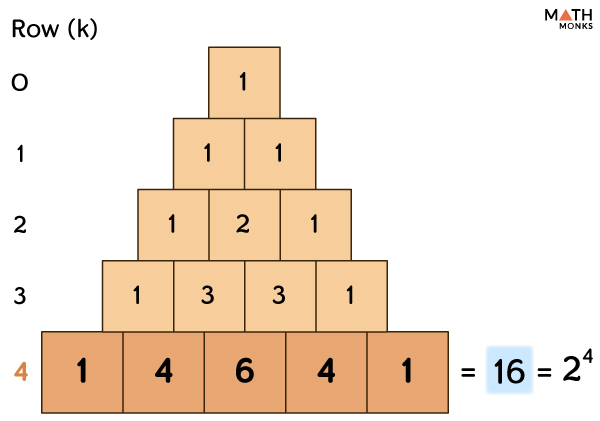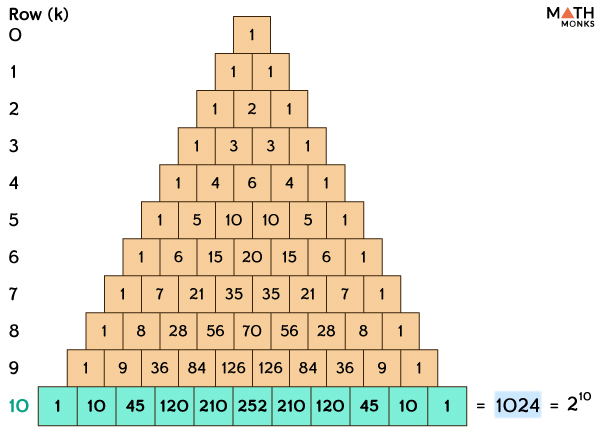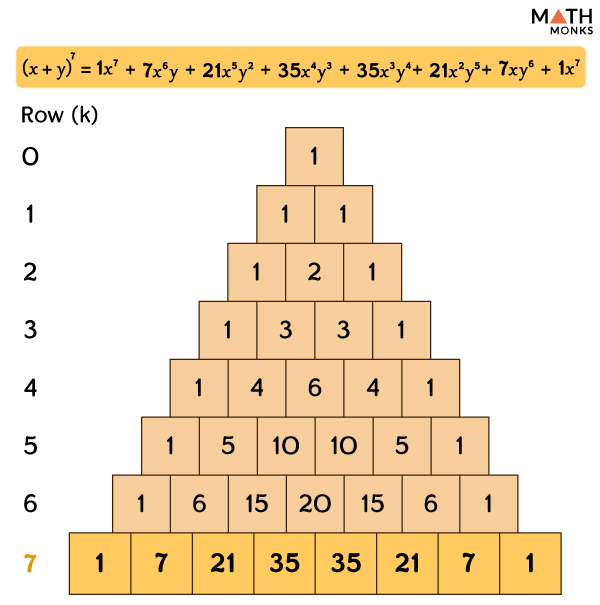# Pascal’s Triangle

Pascal’s triangle is a number triangle that starts with 1 on the top and continues such that each row has 1 at its two ends. It is named after Blaise Pascal, a 17th-century famous French mathematician and philosopher.

## How to Make Pascal’s Triangle

We consider the left-most element in each row as the 0th element. Then the element next to the right of the 0th element is the 1st element. Similarly, the element next to the 1st element is the 2nd element, and so on.

In Pascal’s triangle, the middle numbers are filled such that it is the sum of those adjacent numbers placed just above it.

We consider the adjacent elements to 1 in every row as 0 because there is nothing next to 1 in each row. We repeat this method to construct each subsequent row.

The same process is repeated to fill up the other elements as the Pascal’s triangle has infinite rows.

## Patterns

Pascal’s triangle has different patterns within the triangle. Some of them are listed:

### Diagonals

• The first diagonal is ‘1’
• The next diagonal contains the counting or natural numbers (1, 2, 3,……)
• The third diagonal contains the triangular numbers (1, 3, 6, 10, 15,……)
• The fourth diagonal contains tetrahedral numbers (1, 4, 10, 20,……)
• The fifth diagonal contains pentatope numbers (1, 5, 15, 35,……) and so on.

### Symmetrical

In Pascal’s triangle, the numbers on the left side are identical to those on the right, just like a mirror image. Thus the triangle is symmetrical.

### Horizontal Sums

If we add the numbers in each row, the value doubles each time compared to the previous row. The sums are all powers of 2 and thus can be represented as 2k for the kth row, k = 0, 1, 2, 3,……

We write the summation identity as ${\sum ^{k}_{n=0}\begin{pmatrix} k \\ n \end{pmatrix}}$ = 2kFind the sum of all elements in the 4th row of Pascal’s Triangle.

Solution:As we know,
${\sum ^{k}_{n=0}\begin{pmatrix} k \\ n \end{pmatrix}}$ =2k
Here, the sum of all elements in the 4th row will be,
24  = ${\sum ^{4}_{n=0}\begin{pmatrix} 4 \\ n \end{pmatrix}}$ = ${\begin{pmatrix} 4 \\ 0 \end{pmatrix}}$ + ${\begin{pmatrix} 4 \\ 1 \end{pmatrix}}$ + ${\begin{pmatrix} 4 \\ 2 \end{pmatrix}}$ + ${\begin{pmatrix} 4 \\ 3 \end{pmatrix}}$ + ${\begin{pmatrix} 4 \\ 4 \end{pmatrix}}$
= 1+ 4 + 6 + 4 + 1 = 16

Work out the sum of all elements in the 10th row of Pascal’s Triangle.

Solution:As we know,
${\sum ^{k}_{n=0}\begin{pmatrix} k \\ n \end{pmatrix}}$ = 2k
Here, the sum of all elements in the 10th row will be,
210 = ${\sum ^{10}_{n=0}\begin{pmatrix} 10 \\ n \end{pmatrix}}$ = ${\begin{pmatrix} 10 \\ 0 \end{pmatrix}}$ + ${\begin{pmatrix} 10 \\ 1 \end{pmatrix}}$ + ${\begin{pmatrix} 10 \\ 2 \end{pmatrix}}$ + ${\begin{pmatrix} 10 \\ 3 \end{pmatrix}}$ + ${\begin{pmatrix} 10 \\ 4 \end{pmatrix}}$ + ${\begin{pmatrix} 10 \\ 5 \end{pmatrix}}$ + ${\begin{pmatrix} 10 \\ 6 \end{pmatrix}}$ + ${\begin{pmatrix} 10 \\ 7 \end{pmatrix}}$ + ${\begin{pmatrix} 10 \\ 8 \end{pmatrix}}$ + ${\begin{pmatrix} 10 \\ 9 \end{pmatrix}}$ + ${\begin{pmatrix} 10 \\ 10 \end{pmatrix}}$
= 1 + 10 + 45 + 120 + 210 + 252 + 210 + 120 + 45 + 10 + 1 = 1024

### Exponents of 11

Each line of Pascal’s triangle is the powers or the exponents of 11.

• 11⁰ = 1 (the first row contains only 1)
• 11¹ = 11 (the second row has 1 and 1)
• 11² = 121 (the third row has 1, 2, 1) and so on.

But for 11⁵, how can we relate to the above pattern?

Here, the digits overlap, like shown below.

The same thing happens with 11⁶ too.

The rest of the triangle follows the same pattern.

### Square Property

In the second diagonal of Pascal’s triangle, the square of a number is equal to the sum of the numbers next to it and below both of them.

For example,

3 (from third diagonal) + 6 (from third diagonal) = 9 = 32 (3 from second diagonal)

### Fibonacci Sequence

We can obtain a Fibonacci series in Pascal’s Triangle by summing the different diagonal elements of the triangle as shown.

### Odds and Evens

Suppose we shade the even and odd elements of Pascal’s triangle (marked in different colors). In that case, we will get a pattern similar to the Sierpinski Triangle.

### Paths

In Pascal’s Triangle, each entry represents the number of paths from the top down.

For example, there is only one path from the top down to any 1, two different paths to 2, and 3 pathways to 3.

### Prime Numbers in the Triangle

Another exciting pattern visible in the triangle deals with prime numbers. Suppose a row begins with a prime number or is a prime-numbered row. Then, all the numbers in that row except the 1’s are divisible by that prime number. Looking at the 7th row (1 7 21 35 35 21 7 1), we can see that 7, 21, and 35 all are divisible by 7.

However, this property does not hold true for the composite numbers. For example, if we look at row 8 (1 8 28 56 70 56 28 8 1), we can see that 28 and 70 are not divisible by 8 (see example 2 diagram).

## The Formula for Any Entry in Pascal’s Triangle

To find the value of any element, say the jth element of kth row of Pascal’s triangle, we use the formula:

xk,j= ${\begin{pmatrix} k \\ j \end{pmatrix}}$

Here ${\begin{pmatrix} k \\ j \end{pmatrix}}$ =${\begin{pmatrix} k-1 \\ j-1 \end{pmatrix}}$ +${\begin{pmatrix} k-1 \\ j \end{pmatrix}}$ and j is any non negative integer such that 0${\leq}$j${\leq}$k

The above formula is also written as:

${\begin{pmatrix} k \\ j \end{pmatrix}}$ (i.e. , k choose j) = C(k,j) =kCj = ${\dfrac{k!}{j!\left( k-j\right) !}}$

Here = kCj denotes the jth element in the kth row.

The elements of Pascal’s Triangle is also be written as: (illustration is given up to row 7, starting from row 0)

Find the 3rd element of the 5th row in Pascal’s triangle.

Solution:

As we know,
kCj =  ${\begin{pmatrix} k \\ j \end{pmatrix}}$ =${\begin{pmatrix} k-1 \\ j-1 \end{pmatrix}}$  + ${\begin{pmatrix} k-1 \\ j \end{pmatrix}}$ =  k-1Cj-1k-1Cj
Here k = 5 and j = 3 so, we have to calculate 5C3
Using the above formula we get 5C3 = (5-1)C(3-1) + (5-1)C3=4C2+ 4C3= 2ndelement of 4th row + 3rd element of 4th row =6 + 4 =10 .

## Pascal’s Triangle Binomial Expansion

In Pascal’s Triangle, each number represents the coefficient of the terms of binomial expansion (x+y)n, where x and y are any two variables and n = 0,1,2,……..

Now expanding (x+y)n, we get,

(x+y)n = a0xn + a1x(n-1)y + a2x(n-2)y2+………..+a(n-1)xy(n-1) + anyn

Here, the coefficients of the form ak, k = 0,1,2,…..n are the elements in the nth row of Pascal’s Triangle and can be expressed as ak =${\begin{pmatrix} n \\ k \end{pmatrix}}$ =nCk =(n-1)C(k-1) + (n-1)Ck

For example, if we expand the expression (x+y)n for n=4,

(x+y)4 =a0x4 + a1x3y + a2x2y2 + a3xy3 + a4y4

= 4C0x4 + 4C1x3y +4C2x2y2 +4c3xy3 + 4C4y4

=(1) x4 + (4)x3y + (6)x2y2 + (4)xy3 + (1)y4

Here the coefficients 1, 4, 6, 4, 1 represent elements in the 4th row.

Find all the coefficients of the expansion of the polynomial (x+y)7 using Pascal’s triangle.

Solution:The coefficients of the expansion of the (x+y)7 will actually be the elements in row 7 of Pascal’s triangle.
Elements in the 7th row of Pascal’s Triangle are 1, 7, 21, 35, 35, 21, 7,1 .
So the coefficients of the expansion of (x+y)7 are 1, 7, 21, 35, 35, 21, 7,1

## How to Use the Pascal’s Triangle

Pascal’s triangle has several real-world applications, such as in mathematical calculations, including probability, combinations, etc.

### Pascal’s Triangle Probability

Pascal’s triangle is also used to find various probability conditions. For example, if we toss a coin just once, there are only two possible outcomes, either Head (H) or Tail (T).

If we toss it two times, there is one possibility of getting both heads HH or tails TT, but there are two possibilities of getting at least a head or tail, i.e., HT or TH.

Now if we consider Pascal’s Triangle, we can see the table based on the number of tosses and outcomes given below:

Find the probability of getting exactly two heads when a coin is tossed four times.

Solution:

As we know,
${\sum ^{k}_{n=0}\begin{pmatrix} k \\ n \end{pmatrix}}$ = 2k, for kth row,  k = 0, 1, 2, 3,……
Now we know that the number of times a coin is tossed = Row of Pascal’s Triangle
So,  the number of total outcomes = 24 = 16 = (1 + 4 + 6 + 4 + 1), where 6 out of them give exactly two heads. So, the probability of getting exactly two heads is 6/16 or 37.5%.

### Combinations

Pascal’s triangle also shows the different ways by which we can combine its various elements.

The number of ways r number of objects is chosen out of n objects irrespective of any order and  repetition is given by:

nCr= ${\dfrac{n!}{r!\left( n-r\right) !}}$, which is the rth element of the nth row of Pascal’s Triangle.

Suppose we have 15 pool balls. How many ways can we choose just 5 of them (ignoring the order in which we select them)?

Here, n = 15, r = 5

If we go down to the start of row 15 and then along 5 places to the right, we find the answer 3003, which is the same if we calculate 15C5 separately.

### Hockey Stick Identity

In Pascal’s triangle, let us start at any of the ‘1’ elements (apart from the topmost row) and then move on the left or right side along the row.

Suppose we sum the elements diagonally in a straight line and stop anywhere. In that case, we will find that the next element down diagonally in the opposite direction will equal the sum. When we circle these elements in the triangle, these create a shape like a ‘hockey stick’.

If we begin at the pth row and end on the qth row, the sum will be

${\sum ^{q}_{k=p}\begin{pmatrix} k \\ p \end{pmatrix}}$  = ${\begin{pmatrix} q+1 \\ p+1 \end{pmatrix}}$

Find ${\sum ^{6}_{k=3}\begin{pmatrix} k \\ 3 \end{pmatrix}}$ for Pascal’s  Triangle.

Solution:As we know, that by Hockey Stick identity
${\sum ^{q}_{k=p}\begin{pmatrix} k \\ p \end{pmatrix}}$  = ${\begin{pmatrix} q+1 \\ p+1 \end{pmatrix}}$, here p = 3 and q = 6
Here, we begin at the 3rd row and end at the 6th row,
${\sum ^{6}_{k=3}\begin{pmatrix} k \\ 3 \end{pmatrix}}$ = ${\begin{pmatrix} 6+1 \\ 3+1 \end{pmatrix}}$ =${\begin{pmatrix} 7 \\ 4 \end{pmatrix}}$ =35

Also, let’s look at the diagram, summing the elements starting from 1 on the 3rd row and going diagonally down in a straight line up to the 6th row. We can see 1 + 4 + 10 + 20 = 35, the next element down diagonally in the opposite direction.